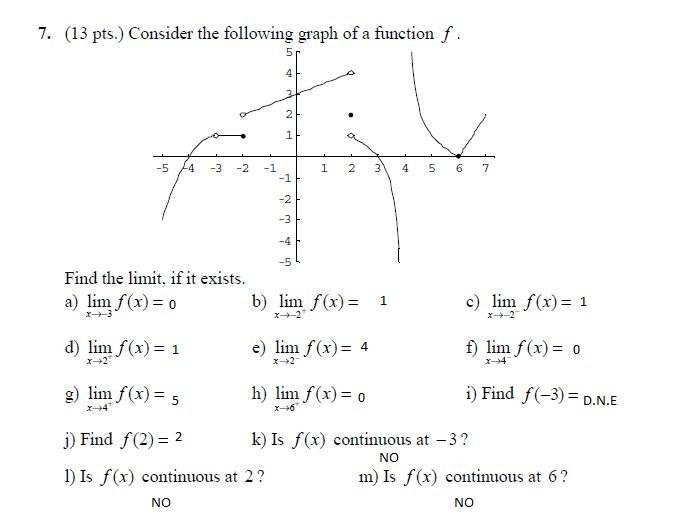# Math Help Forum: Find the limit if it exists

calculus123
I'm having a really difficult time understanding limits from the graph. I don't completely understand how to find if the limit exists or not. I tried to do these for my homework but I don't know if they are right. Could someone please help me understand limits?Homework Helper
Gold Member
I'm having trouble reading the little + and - symbols in your limits. Is there any way you can display this at a higher resolution?

 just noticed that in b and c, the limits are as x approaches -2, whereas in d and e, x approaches 2.

The first one that looks wrong is b. Is that the limit as x approaches -2 from the positive side? If so, the answer is not 1. To answer this question, ignore everything to the left of x = -2, and ignore what happens at x = -2 itself. Focus only on what the graph is doing as x approaches -2 from the right side. What number is the graph approaching?

utstatistics
B and C are -2, whereas D and E are +2. Going blind looking at it.

Staff Emeritus
Homework Helper
The limits appear to be:
\begin{align*}
\lim_{x \to -3} f(x) \\
\lim_{x \to -2^+} f(x) \\
\lim_{x \to -2^-} f(x) \\
\lim_{x \to 2^+} f(x) \\
\lim_{x \to 2^-} f(x) \\
\lim_{x \to 4^-} f(x) \\
\lim_{x \to 4^+} f(x) \\
\lim_{x \to 6^+} f(x)
\end{align*}

Staff Emeritus